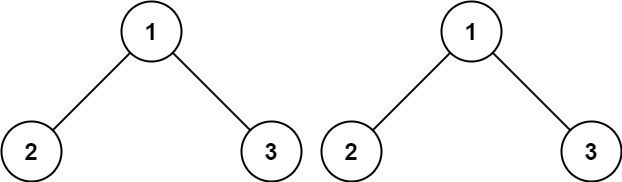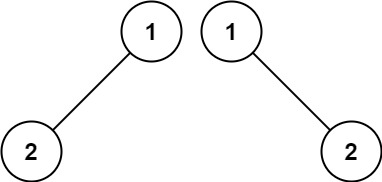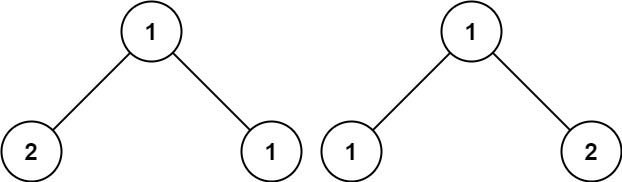# 100.same-tree

## Statement

• Difficulty: Easy
• Tag: `树` `深度优先搜索` `广度优先搜索` `二叉树```````输入：p = [1,2,3], q = [1,2,3]

````````````输入：p = [1,2], q = [1,null,2]

````````````输入：p = [1,2,1], q = [1,1,2]

``````

• 两棵树上的节点数目都在范围 `[0, 100]`
• `-104 <= Node.val <= 104`

• Difficulty: Easy
• Tag: `Tree` `Depth-First Search` `Breadth-First Search` `Binary Tree`

Given the roots of two binary trees `p` and `q`, write a function to check if they are the same or not.

Two binary trees are considered the same if they are structurally identical, and the nodes have the same value.

Example 1:``````Input: p = [1,2,3], q = [1,2,3]
Output: true
``````

Example 2:``````Input: p = [1,2], q = [1,null,2]
Output: false
``````

Example 3:``````Input: p = [1,2,1], q = [1,1,2]
Output: false
``````

Constraints:

• The number of nodes in both trees is in the range `[0, 100]`.
• `-104 <= Node.val <= 104`

## Solution

``````# Definition for a binary tree node.
# class TreeNode:
#     def __init__(self, val=0, left=None, right=None):
#         self.val = val
#         self.left = left
#         self.right = right
class Solution:
def dfs(self, l: TreeNode, r: TreeNode) -> bool:
if not l and not r:
return True

if not l or not r:
return False

if l.val != r.val:
return False

return self.dfs(l.left, r.left) and self.dfs(l.right, r.right)

def isSameTree(self, p: TreeNode, q: TreeNode) -> bool:
return self.dfs(p, q)
``````